# Greedy MCQ - 1

## 10 Questions MCQ Test RRB JE for Computer Science Engineering | Greedy MCQ - 1

Description
This mock test of Greedy MCQ - 1 for Computer Science Engineering (CSE) helps you for every Computer Science Engineering (CSE) entrance exam. This contains 10 Multiple Choice Questions for Computer Science Engineering (CSE) Greedy MCQ - 1 (mcq) to study with solutions a complete question bank. The solved questions answers in this Greedy MCQ - 1 quiz give you a good mix of easy questions and tough questions. Computer Science Engineering (CSE) students definitely take this Greedy MCQ - 1 exercise for a better result in the exam. You can find other Greedy MCQ - 1 extra questions, long questions & short questions for Computer Science Engineering (CSE) on EduRev as well by searching above.
QUESTION: 1

Solution:
QUESTION: 2

Solution:
QUESTION: 3

### A networking company uses a compression technique to encode the message before transmitting over the network. Suppose the message contains the following characters with their frequency: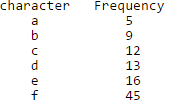Note : Each character in input message takes 1 byte. If the compression technique used is Huffman Coding, how many bits will be saved in the message?

Solution:

Total number of characters in the message = 100.
Each character takes 1 byte. So total number of bits needed = 800.

After Huffman Coding, the characters can be represented with:
f: 0
c: 100
d: 101
a: 1100
b: 1101
e: 111

Total number of bits needed = 224
Hence, number of bits saved = 800 - 224 = 576
See here for complete explanation and algorithm.

QUESTION: 4

What is the time complexity of Huffman Coding?

Solution:

O(nlogn) where n is the number of unique characters. If there are n nodes, extractMin() is called 2*(n – 1) times. extractMin() takes O(logn) time as it calles minHeapify(). So, overall complexity is O(nlogn). See here for more details of the algorithm.

QUESTION: 5

In question #2, which of the following represents the word "dead"?

Solution:

The Huffman Tree generated is: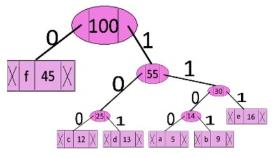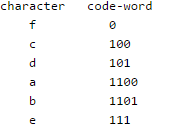The word dead can be represented as: 101 111 1100 101 However, the alternative codeword can also be found by assigning 1 to the left edge and 0 to the right edge of the tree, i.e. dead can also be represented as: 010 000 0011 010 See here for more details of the algorithm.

QUESTION: 6

Which of the following is true about Kruskal and Prim MST algorithms? Assume that Prim is implemented for adjacency list representation using Binary Heap and Kruskal is implemented using union by rank.

Solution:
QUESTION: 7

Which of the following is true about Huffman Coding.

Solution:

Huffman coding is a lossless data compression algorithm. The codes assigned to input characters are Prefix Codes, means the codes are assigned in such a way that the code assigned to one character is not prefix of code assigned to any other character. This is how Huffman Coding makes sure that there is no ambiguity when decoding.

QUESTION: 8

Suppose the letters a, b, c, d, e, f have probabilities 1/2, 1/4, 1/8, 1/16, 1/32, 1/32 respectively. Which of the following is the Huffman code for the letter a, b, c, d, e, f?

Solution:

We get the following Huffman Tree after applying Huffman Coding Algorithm. The idea is to keep the least probable characters as low as possible by picking them first.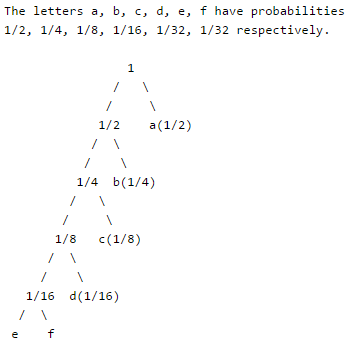QUESTION: 9

Suppose the letters a, b, c, d, e, f have probabilities 1/2, 1/4, 1/8, 1/16, 1/32, 1/32 respectively. What is the average length of Huffman codes?

Solution:

We get the following Huffman Tree after applying Huffman Coding Algorithm. The idea is to keep the least probable characters as low as possible by picking them first.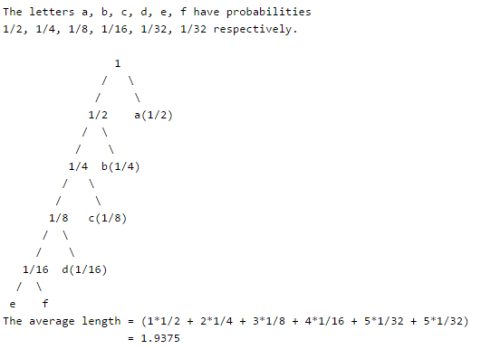QUESTION: 10

Consider the undirected graph below: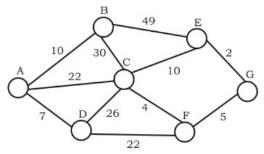Q.
Using Prim's algorithm to construct a minimum spanning tree starting with node A, which one of the following sequences of edges represents a possible order in which the edges would be added to construct the minimum spanning tree?

Solution:

A and B are False  : The idea behind Prim’s algorithm is to construct a spanning tree - means all vertices must be connected but here vertices are disconnected C. False. Prim's is a greedy algorithm and At every step, it considers all the edges that connect the two sets, and picks the minimum weight edge from these edges. In this option weight of AB<AD so must be picked up first. D.TRUE. It represents a possible order in which the edges would be added to construct the minimum spanning tree. Prim’s algorithm is also a Greedy algorithm. It starts with an empty spanning tree. The idea is to maintain two sets of vertices. The first set contains the vertices already included in the MST, the other set contains the vertices not yet included. At every step, it considers all the edges that connect the two sets, and picks the minimum weight edge from these edges. After picking the edge, it moves the other endpoint of the edge to the set containing MST.